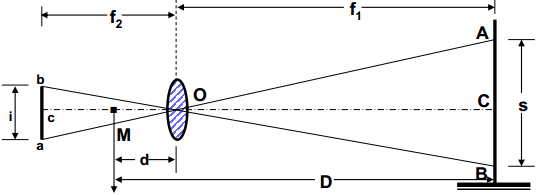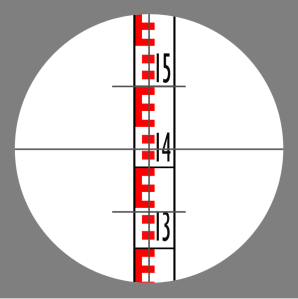# Tacheometric Surveying- Methods, Detail Procedures

Tacheometry is a branch of angular surveying in which the horizontal and vertical distances are obtained by optical means as opposed to the ordinary process of chain and tape. This is done with the help of two special type of instruments- transit theodolite and stadia rod. On the other hand, other conventional surveying methods like chain surveying or traverse surveying need the surveyor to take a linear measurement on the field by a tape or a chain. These are relatively slower processes and also tiresome.

### Tacheometric Surveying Instruments

Tacheometric Surveying is done with the help of Tacheometer and Stadia Rod. To read details of the tacheometric surveying instruments click the following link:

### Different Methods of Tacheometric Measurements

The various methods of the tacheometric survey may be classified as follows:

1. Fixed Hair Method
2. Movable Hair Method, or Subtense Method
2. The Tangential System
3. Measurements by means of Special Instruments

A brief description of these methods is given below.

#### Fixed Hair Method

• In this method, the angle at the instrument at A subtended by a known short distance along a staff kept at B is made with the help of a stadia diaphragm having stadia wires at fixed or constant distance apart.
• The readings are on the staff corresponding to all the three wires taken.
• The staff intercept which means the difference of the readings corresponding to the top and bottom stadia wires will, therefore, depend on the distance of the stadia/level staff from the tacheometer
• When the staff intercept is more than the length of the staff, the only half intercept is read.
• This is the most common method is tacheometry and the same ‘stadia method’ generally bears reference to this method.

#### Subtense Method

• This method is almost same as the stadia method except that the stadia interval is variable.
• A suitable arrangement is made to vary the distance between the stadia hair as to set them against the two targets on the staff kept at the point under observation.
• Thus, in this case, the staff intercept, i.e., the distance between the two targets is kept fixed while the stadia interval, i.e., the distance between the stadia hair is variable.
• As in the case of fixed hair method, inclined sights may also be taken.

As in the field of tacheometric surveying ‘Stadia Method’ is the most widely used procedure so we will discuss the principle behind it. The stadia method follows the principle that in similar isosceles triangles the ratio of the perpendicular to the base is constant.

In fig. let two rays be equally inclined to the central ray. Here central ray is shown as OC. A2B2, A1B1, and AB are staff intercepts i.e difference between upper and lower stadia reading.

Evidently, OC2/A2B2= OC1/A1B1= OC/AB= constant K= 0.5cot (β/2)

This constant depends entirely on the angle β. Let, the constant is found to be 100. It means the distance between the staff and the point O will be 100 times the staff intercept.

#### The Distance-Elevation Formulae For Horizontal SightSuppose,

the interval between stadia hairs is given by i=ab,
staff intercept is s,
f is the focal length of the objective,
D is the horizontal distance of the staff from the vertical axis of the instruments.

The horizontal distance between the axis and the staff is given by the following equation

D = f1 + d= f1= (s/i)*f+(f+d)

This is the distance equation. Staff intercept is found by subtracting the reading of the upper and lower stadia reading.

The constant k = f/i is called the multiplying constant or stadia interval factor and the constant (f + d) =C is known as the additive constant of the tacheometer but the latter one is made zero by using an anallatic lens in the instrument.

### Determination of Tacheometric Constants on FieldIn most cases, we do not really know the value of f (focal length of the objective) so we have to determine the constant k and C on the field with a different approach as below:

1. Measure a line (about 100m long) on the fairly level ground and drive pegs at some interval, say 50 meters.
2. Keep the staff on the previously determined station and observe the corresponding staff intercepts (upper and lower stadia reading) with horizontal sight.
3. Knowing the values of D and s for different points, a number of simultaneous equations can be formed by substituting the values of D and s in equation D = k.s + C. The simultaneous solution of successive pairs will give the values of k and C, and the average of these can be found.

### Disclaimer

Please note that the information in Civiltoday.com is designed to provide general information on the topics presented. The information provided should not be used as a substitute for professional services.